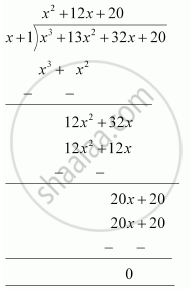# Factorise :- x^3 + 13x^2 + 32x + 20 - Mathematics

Factorise :- x3 + 13x2 + 32x + 20

#### Solution

Let p(x) = x3 + 13x2 + 32x + 20

All the factors of 20 have to be considered. Some of them are ±1,

± 2, ± 4, ± 5 ……

By trial method,

p(−1) = (−1)3 + 13(−1)2 + 32(−1) + 20

= − 1 +13 − 32 + 20

= 33 − 33 = 0

As p(−1) is zero, therefore, + 1 is a factor of this polynomial p(x).

Let us find the quotient on dividing x3 + 13x2 + 32x + 20 by (x + 1).

By long division,It is known that,

Dividend = Divisor × Quotient + Remainder

x3 + 13x2 + 32x + 20 = (+ 1) (x2 + 12x + 20) + 0

= (+ 1) (x2 + 10x + 2+ 20)

= (x + 1) [x (+ 10) + 2 (+ 10)]

= (x + 1) (+ 10) (+ 2)

= (x + 1) (x + 2) (x + 10)

Concept: Factorising the Quadratic Polynomial (Trinomial) of the type ax2 + bx + c, a ≠ 0.
Is there an error in this question or solution?

#### APPEARS IN

NCERT Class 9 Maths
Chapter 2 Polynomials
Exercise 2.4 | Q 5.3 | Page 44